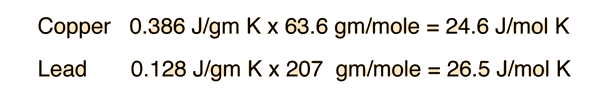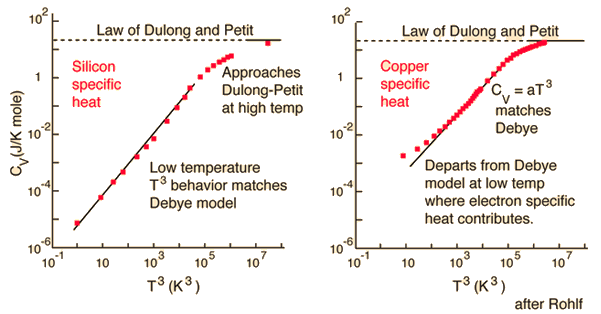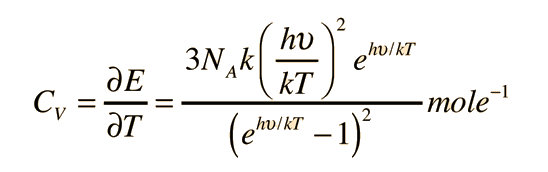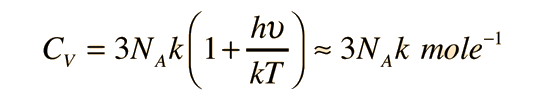# Law of Dulong and Petit

The specific heat of copper is 0.093 cal/gm K (.389 J/gm K) and that of lead is only 0.031 cal/gm K(.13 J/gm K). Why are they so different? The difference is mainly because it is expressed as energy per unit mass; if you express it as energy per mole, they are very similar. It is in fact that similarity of the molar specific heats of metals which is the subject of the Law of Dulong and Petit. The similarity can be accounted for by applying equipartition of energy to the atoms of the solids.

From just the translational degrees of freedom you get 3kT/2 of energy per atom. Energy added to solids takes the form of atomic vibrations and that contributes three additional degrees of freedom and a total energy per atom of 3kT. The specific heat at constant volume should be just the rate of change with temperature (temperature derivative) of that energy.When looked at on a molar basis, the specific heats of copper and lead are quite similar:Define constants Table of specific heats
 Why is there a departure from the Law of Dulong and Petit at low temperatures?
 Why don't the electrons contribute to the specific heat?
Index

 HyperPhysics***** Thermodynamics R Nave
Go Back

# Departure from the Law of Dulong and Petit

The Law of Dulong and Petit is based on Maxwell-Boltzmann statistics, and for low temperatures, quantum statistics must be used.Explaining the drastic departure from the Law of Dulong and Petit was a major contribution of Einstein and Debye.Index

Reference
Rohlf
Ch 14.

 HyperPhysics***** Thermodynamics R Nave
Go Back

# Einstein's Contribution to Specific Heat Theory

The Law of Dulong and Petit assumed that Maxwell-Boltzmann statistics and equipartition of energy could be applied even at low temperatures. Einstein recognized that for a quantum harmonic oscillator at energies less than kT, the Einstein-Bose statistics must be applied. This was the same conclusion that was drawn about blackbody radiation. The statistical distribution of energy in the vibrational states gives average energy:where this frequency is the frequency of a quantum vibrator. There are three degrees of freedom per vibrator, so the total energy isThe derivative of this gives:For high temperatures, this expression approaches agreement with the Law of Dulong and Petit.

 Show

In the Einstein treatment, the appropriate frequency in the expression had to be determined empirically by comparison with experiment for each element. The quantity hu/k is sometimes called the Einstein temperature. Although the general match with experiment was reasonable, it was not exact. Debye advanced the treatment by treating the quantum oscillators as collective modes in the solid which are now called "phonons".

 Refinement by Peter Debye
Index

Reference
Blatt
Sec 4.3.

Rohlf
Ch 14

 HyperPhysics***** Thermodynamics R Nave
Go Back

# The High Temperature Limit of the Einstein Specific Heat

Einstein's introduction of quantum behavior showed why the specific heat became temperature dependent at low temperatures, and it had a high temperature limit which agreed with the Law of Dulong and Petit. To show this, note that for high temperatures, a series expansion of the exponential givesThe Einstein specific heat expression then becomesThis reduces to the Law of Dulong and Petit.Index

References
Rohlf
Ch 14

Blatt
Sec 4.3.

 HyperPhysics***** Thermodynamics R Nave
Go Back